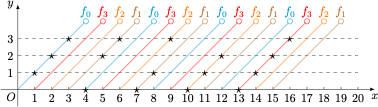# 每日一题穿肉串

$[x]$ 表示取数 $x$ 的整数部分，例如 $\left[\dfrac{15}{4}\right]=3$ 等，若$y=4\left(\frac{x+[u]}{4}-\left[\frac{x+[u]}{4}\right]\right),$且$\begin{array}{c|c|c|c|c}\hline x&1,8,11,14& 2,5,12,15&3,6,9,16& 4,7,10,13\\ \hline y&1&2&3&0 \\ \hline\end{array}$ 则表达式中 $u$ 等于_______．### 《每日一题穿肉串》有1条回应

1.louxin2020说：

u的表达式应该是不唯一的